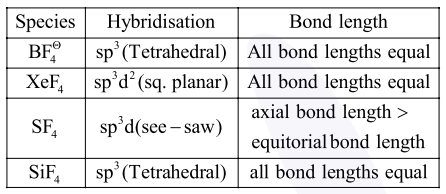# Which among the following species`
Question:

Which among the following species has unequal bond lengths ?

1. $\mathrm{BF}_{4}^{-}$

2. $\mathrm{XeF}_{4}$

3. $\mathrm{SF}_{4}$

4. $\mathrm{SiF}_{4}$

Correct Option: , 3

Solution: